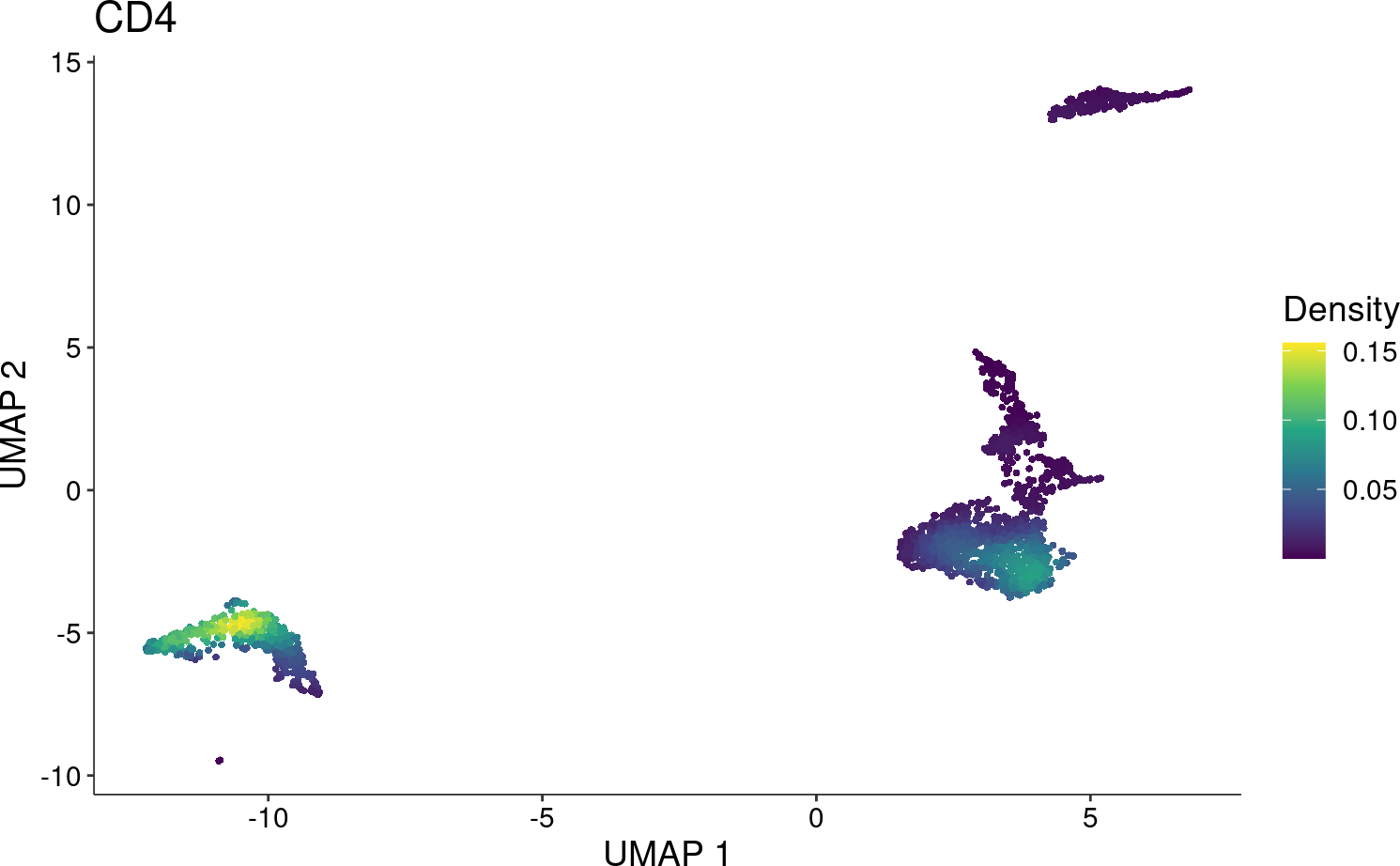1 Overview

Due to the sparsity observed in single-cell data (e.g. RNA-seq, ATAC-seq), the visualization of cell features (e.g. gene, peak) is frequently affected and unclear, especially when it is overlaid with clustering to annotate cell types. Nebulosa is an R package to visualize data from single cells based on kernel density estimation. It aims to recover the signal from dropped-out features by incorporating the similarity between cells allowing a “convolution” of the cell features.

2 Import libraries

For this vignette, let’s use Nebulosa with the scran and scater packages. First, we’ll do a brief/standard data processing.

library("Nebulosa")
library("scater")
library("scran")
library("DropletUtils")
library("BiocFileCache")

3 Data pre-processing

Let’s download a dataset of 3k PBMCs (available from 10X Genomics). For the purpose of this vignette, let’s use the BiocFileChache package to dowload the data and store it in a temporary directory defined by the tempdir() function. To import the count data, we’ll use the read10xCounts from the DropletUtils package.

data_file <- bfcrpath(bfc, file.path(
"https://s3-us-west-2.amazonaws.com/10x.files/samples/cell",
"pbmc3k",
"pbmc3k_filtered_gene_bc_matrices.tar.gz"
))
untar(data_file, exdir = tempdir())
"filtered_gene_bc_matrices",
"hg19"
))

The default feature names are Ensembl ids, let’s use thegene names and set them as row names of the sce object. The following step will use the gene names as rownames and make them unique by appending it’s corresponding Ensemble id when a gene-name duplicate is found.

rownames(pbmc) <- uniquifyFeatureNames(rowData(pbmc)[["ID"]],
rowData(pbmc)[["Symbol"]])

4 Quality control

First, let’s remove features that are not expressed in at least 3 cells.

i <- rowSums(counts(pbmc) > 0)
is_expressed <- i > 3
pbmc <- pbmc[is_expressed, ]

And cells not expressing at least one UMI in at least 200 genes.

i <- colSums(counts(pbmc) > 0)
is_expressed <- i > 200
pbmc <- pbmc[,is_expressed]

Finally, let’s remove outlier cells based on the number of genes being expressed in each cell, library size, and expression of mitochondrial genes using the perCellQCMetrics and quickPerCellQC functions from the scater package.

is_mito <- grepl("^MT-", rownames(pbmc))
qcstats <- perCellQCMetrics(pbmc, subsets = list(Mito = is_mito))
qcfilter <- quickPerCellQC(qcstats, percent_subsets = c("subsets_Mito_percent"))

4.1 Data normalization

Let’s normalize the data by scaling the counts from each cell across all genes by the sequencing depth of each cell and using a scaling factor of 1 x 10^4. Then, we can stabilize the variance by calculating the pseudo-natural logarithm using the log1p function.

logcounts(pbmc) <- log1p(counts(pbmc) / colSums(counts(pbmc)) * 1e4)

Please refer to the OSCA website for more details on other normalization strategies: https://osca.bioconductor.org/normalization.html

4.2 Dimensionality reduction

A reduced set of variable genes are expected to drive the major differences between the cell populations. To identify these genes, let’s use the modelGeneVar() and getTopHVGsfrom() from scran by selecting the top 3000 most highly-variable genes.

dec <- modelGeneVar(pbmc)
top_hvgs <- getTopHVGs(dec, n = 3000)

Once the data is normalized and highly-variable features have been determined, we can run a Principal Component Analysis (PCA) to reduce the dimensions of our data to 50 principal components. Then, we can run a Uniform Manifold Approximation and Projection (UMAP) using the principal components to obtain a two-dimensional representation that could be visualized in a scatter plot.

set.seed(66)
pbmc <- runPCA(pbmc, scale = TRUE, subset_row = top_hvgs)

Finally, we can run the UMAP as follows:

pbmc <- runUMAP(pbmc, dimred = "PCA")

4.3 Clustering

To assess cell similarity, let’s cluster the data by constructing a Shared Nearest Neighbor (SNN) Graph using the first 50 principal components and applying cluster_louvain() from the igraph package.

g <- buildSNNGraph(pbmc, k = 10, use.dimred = "PCA")
clust <- igraph::cluster_louvain(g)\$membership
colLabels(pbmc) <- factor(clust)

5 Visualize data with Nebulosa

The main function from Nebulosa is the plot_density.

Let’s plot the kernel density estimate for CD4 as follows

plot_density(pbmc, "CD4")For comparison, let’s also create a standard scatter plot using scater

plotUMAP(pbmc, colour_by = "CD4")